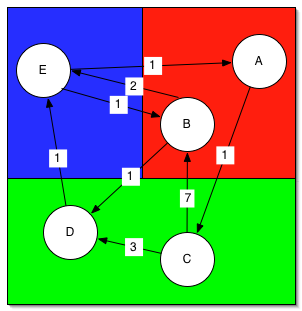#Boost C++ Libraries

...one of the most highly regarded and expertly designed C++ library projects in the world.

# Parallel Shortest Paths

To illustrate the use of the Parallel Boost Graph Library, we illustrate the use of both the sequential and parallel BGL to find the shortest paths from vertex A to every other vertex in the following simple graph:With the sequential BGL, the program to calculate shortest paths has three stages. Readers familiar with the BGL may wish to skip ahead to the section Distributing the graph.

# Define the graph type

For this problem we use an adjacency list representation of the graph, using the BGL adjacency_list``_ class template. It will be a directed graph (``directedS parameter ) whose vertices are stored in an std::vector (vecS parameter) where the outgoing edges of each vertex are stored in an std::list (listS parameter). To each of the edges we attach an integral weight.

```typedef adjacency_list<listS, vecS, directedS,
no_property,                 // Vertex properties
property<edge_weight_t, int> // Edge properties
> graph_t;
typedef graph_traits < graph_t >::vertex_descriptor vertex_descriptor;
typedef graph_traits < graph_t >::edge_descriptor edge_descriptor;
```

# Construct the graph

To build the graph, we declare an enumeration containing the node names (for our own use) and create two arrays: the first, edge_array, contains the source and target of each edge, whereas the second, weights, contains the integral weight of each edge. We pass the contents of the arrays via pointers (used here as iterators) to the graph constructor to build our graph:

```typedef std::pair<int, int> Edge;
const int num_nodes = 5;
enum nodes { A, B, C, D, E };
char name[] = "ABCDE";
Edge edge_array[] = { Edge(A, C), Edge(B, B), Edge(B, D), Edge(B, E),
Edge(C, B), Edge(C, D), Edge(D, E), Edge(E, A), Edge(E, B)
};
int weights[] = { 1, 2, 1, 2, 7, 3, 1, 1, 1 };
int num_arcs = sizeof(edge_array) / sizeof(Edge);

graph_t g(edge_array, edge_array + num_arcs, weights, num_nodes);
```

# Invoke Dijkstra's algorithm

To invoke Dijkstra's algorithm, we need to first decide how we want to receive the results of the algorithm, namely the distance to each vertex and the predecessor of each vertex (allowing reconstruction of the shortest paths themselves). In our case, we will create two vectors, p for predecessors and d for distances.

Next, we determine our starting vertex s using the vertex operation on the adjacency_list``_ and call ``dijkstra_shortest_paths``_ with the graph ``g, starting vertex s, and two property maps``_ that instruct the algorithm to store predecessors in the ``p vector and distances in the d vector. The algorithm automatically uses the edge weights stored within the graph, although this capability can be overridden.

```// Keeps track of the predecessor of each vertex
std::vector<vertex_descriptor> p(num_vertices(g));
// Keeps track of the distance to each vertex
std::vector<int> d(num_vertices(g));

vertex_descriptor s = vertex(A, g);
dijkstra_shortest_paths
(g, s,
predecessor_map(
make_iterator_property_map(p.begin(), get(vertex_index, g))).
distance_map(
make_iterator_property_map(d.begin(), get(vertex_index, g)))
);
```

# Distributing the graph

The prior computation is entirely sequential, with the graph stored within a single address space. To distribute the graph across several processors without a shared address space, we need to represent the processors and communication among them and alter the graph type.

Processors and their interactions are abstracted via a process group. In our case, we will use MPI for communication with inter-processor messages sent immediately:

```typedef mpi::process_group<mpi::immediateS> process_group_type;
```

Next, we instruct the adjacency_list template to distribute the vertices of the graph across our process group, storing the local vertices in an std::vector:

```typedef adjacency_list<listS,
distributedS<process_group_type, vecS>,
directedS,
no_property,                 // Vertex properties
property<edge_weight_t, int> // Edge properties
> graph_t;
typedef graph_traits < graph_t >::vertex_descriptor vertex_descriptor;
typedef graph_traits < graph_t >::edge_descriptor edge_descriptor;
```

Note that the only difference from the sequential BGL is the use of the distributedS selector, which identifies a distributed graph. The vertices of the graph will be distributed among the various processors, and the processor that owns a vertex also stores the edges outgoing from that vertex and any properties associated with that vertex or its edges. With three processors and the default block distribution, the graph would be distributed in this manner:Processor 0 (red) owns vertices A and B, including all edges outoing from those vertices, processor 1 (green) owns vertices C and D (and their edges), and processor 2 (blue) owns vertex E. Constructing this graph uses the same syntax is the sequential graph, as in the section Construct the graph.

The call to dijkstra_shortest_paths is syntactically equivalent to the sequential call, but the mechanisms used are very different. The property maps passed to dijkstra_shortest_paths are actually distributed property maps, which store properties for local edges or vertices and perform implicit communication to access properties of remote edges or vertices when needed. The formulation of Dijkstra's algorithm is also slightly different, because each processor can only attempt to relax edges outgoing from local vertices: as shorter paths to a vertex are discovered, messages to the processor owning that vertex indicate that the vertex may require reprocessing.